Equilateral triangles are common in geometry courses, so it pays to know about their sides and angles.  We can also calculate their heights and areas once we know the formulas.

So, what do you need to know about equilateral triangles?  Equilateral triangles have 3 sides of the same length and 3 angles with the same measure (60 degrees), but no parallel or perpendicular sides. An equilateral triangle is isosceles and acute, but never scalene or right. Any pair of equilateral triangles is similar, but not necessarily congruent.

Remember that an equilateral triangle is a special type of regular polygon: one with n = 3 sides, interior angles of 60 degrees, and exterior angle of 120 degrees.

Let’s get started.

An equilateral triangle is a triangle where all three sides have the same length.

This gives equilateral certain special properties, which we will discuss below.

### Do Equilateral Triangles Have Equal Angles?

Equilateral triangles do have equal angles.  Since all three sides are the same length, it follows that all three angles have the same measure.

We can calculate the angle as follows: call the angle A.  The three angles in an equilateral triangle all have a measure of A, and the three angles add up to 180 degrees.

This gives us the equation:

• A + A + A = 180
• 3A = 180
• A = 60

So, each angle in an equilateral triangle has a measure of 60 degrees.

### Can Equilateral Triangles Be Isosceles?

An equilateral triangle is always isosceles.  By definition, an isosceles triangle has at least two sides of the same length.

So, an equilateral triangle is a special case of an isosceles triangle (since having three sides of equal length means having at least two sides of equal length).

However, the reverse is not true: not every isosceles triangle is equilateral.  For example, a right isosceles triangle with side lengths 1, 1, and √2 is not equilateral.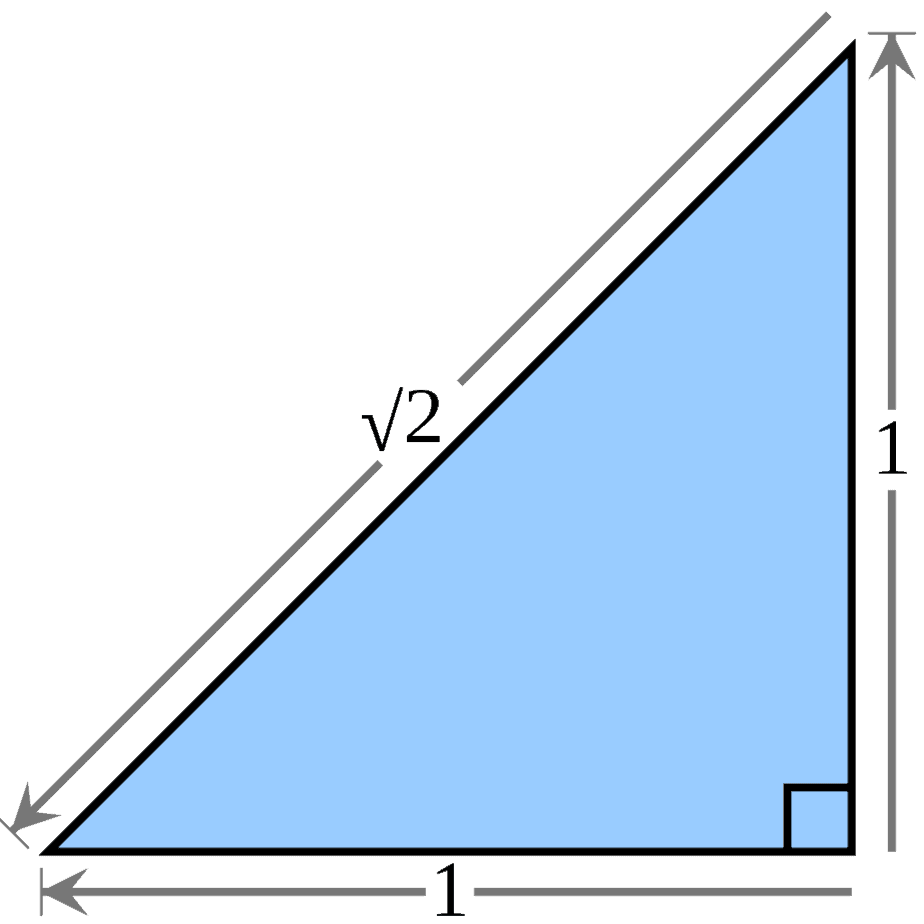This right isosceles triangle has angles 45, 45, and 90, with side lengths 1, 1, and √2, meaning it is not equilateral.

### Can Equilateral Triangles Be Acute?

An equilateral triangle is always acute.  By definition, an acute triangle is one with three acute angles (an acute angle is less than 90 degrees).

Since each of the three angles in an equilateral triangle is 60 degrees, and 60 < 90, this means that all three angles are acute.

However, the reverse is not true: not every acute triangle is equilateral.  For example, an acute triangle with angles 30, 45, 75 is not equilateral – all three sides will have different lengths, since all three angles have different measures.An acute triangle has three acute angles, each of which has a measure less than 90 degrees. An equilateral triangle is acute, but not every acute triangle is equilateral.

### Can Equilateral Triangles Be Scalene?

An equilateral triangle is never scalene.  By definition, a scalene triangle is one where no two sides have the same length (each side has a unique length).

Since an equilateral triangle has the same length for all three sides, it cannot be scalene.  Likewise, no scalene triangle is equilateral.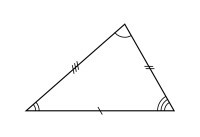A scalene triangle has three distinct side lengths: no pair of sides has the same length. Likewise, a scalene triangle has three distinct angle measures. No scalene triangle is equilateral, and no equilateral triangle is scalene.

### Can An Equilateral Triangle Be A Right Triangle?

An equilateral triangle is never a right triangle.  By definition, a right triangle is one that has a 90 degree angle.

Since an equilateral triangle has three 60 degree angles, it cannot have a 90 degree angle, and so it cannot be a right triangle.  Likewise, no right triangle can be equilateral.A right triangle has a 90 degree angle. This means it cannot be equilateral, since it cannot have three 60 degree angles.

### Are All Equilateral Triangles Similar?

All equilateral triangles are similar.  The reason is that they share the same three angles: they are always 60 degrees.

No matter the side lengths of two equilateral triangles, they are similar because of the shared angles.

### Are All Equilateral Triangles Congruent?

Not all equilateral triangles are congruent.  Remember that congruent is a stronger statement than similar:

• For two triangles to be similar, the three pairs of angles (one from each triangle) must be equal.
• For two triangles to be congruent, they must be similar and the three pairs of sides (one from each angle) must have equal lengths.

So, any two congruent triangles are similar, but two similar triangles may not be congruent.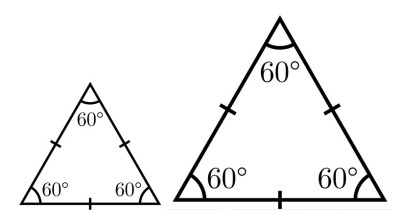These two equilateral triangles are similar (since they have the same angle measures), but they are not congruent (since they have different side lengths).

### Is An Equilateral Triangle A Regular Polygon?

An equilateral triangle is always a regular polygon.  By definition, a regular polygon is a polygon where all angles have the same measure and all sides have the same length (there can be any number of sides and angles).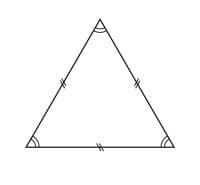An equilateral triangle is a regular polygon with n = 3 sides, interior angles of 60 degrees, and exterior angles of 120 degrees.

For an equilateral triangle, we have a regular polygon with 3 sides (n = 3).

The measure of each interior angle is 180 / 3 = 60 degrees.

The measure of each exterior angle is 360 / 3 = 120 degrees.

### Does An Equilateral Triangle Have Parallel Sides?

An equilateral triangle never has parallel sides.  Remember: any pair of sides in an equilateral triangle will meet (at a 60 degree angle), so any pair of sides cannot be parallel.

Likewise, any polygon with two parallel sides is not a triangle.  Two sides that are parallel would never intersect, so they would never meet to form an angle.

We would need to add and least two more line segments to the figure to “close” the shape.  This would result in a polygon with 4 or more sides, which is not a triangle.

### Does An Equilateral Triangle Have Perpendicular Sides?

An equilateral triangle never has perpendicular sides.  Remember: any pair of sides in an equilateral triangle will meet at a 60 degree angle.  So, no pair of sides can be perpendicular, since perpendicular sides would have to meet at a 90 degree angle.

Likewise, any triangle with two perpendicular sides cannot be equilateral.  When two sides of a triangle are perpendicular, they meet at a 90 degree angle, and so we have a right triangle.  As we mentioned before, a right triangle can never be equilateral.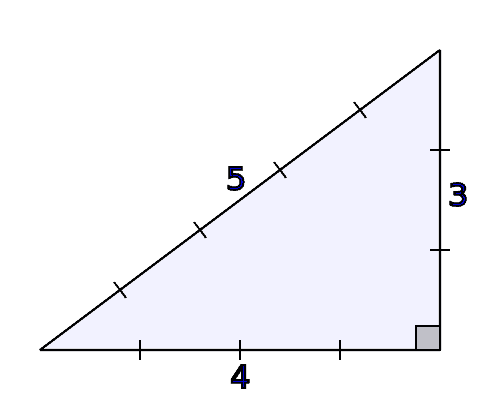A right triangle has perpendicular sides (which meet at the 90 degree angle). This means that a right triangle cannot be equilateral (a 90 degree angle in a right triangle is inconsistent with three 60 degree angles in an equilateral triangle).

### How To Find The Height Of An Equilateral Triangle

The height h of an equilateral triangle with side length x is given by the formula:

• h = (√3 / 2)x

To prove this, we take the following steps:

• First, draw a dotted line from the top corner of the triangle to the opposite side (the dotted line and the opposite side should meet at a 90 degree angle).  This “bisects” the equilateral triangle into two halves.
• Next, look at the right half of the triangle.  This is a 30-60-90 triangle: the perpendicular line gives us 90 degrees, the angle at the far right is still 60 degrees, and the last angle is forced to be 30 degrees (angles add up to 180 in a triangle).  Remember that for this special triangle, the side lengths are in the ratio 1:√3:2.
• Then, set up a ratio of side lengths.  If the side length of the equilateral triangle is x and the height is h, then the ratio is x / h = 2 / √3.
• Finally, solve the ratio to find the height h.  Taking the reciprocal of both sides gives us h / x = √3 / 2, or h = (√3 / 2)x.

Note that we can also use the Pythagorean Theorem to find the height, but the special triangle identity is easier.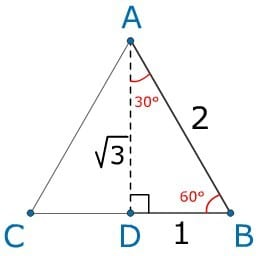We can use the special angles of a 30-60-90 triangle to find the ratios of the side lengths, which are 1:√3:2.

#### Example: How To Find The Height Of An Equilateral Triangle

Let’s say we have an equilateral triangle with a side length of x = 8.  We can use the formula for the height as follows:

• h = (√3 / 2)x
• h = (√3 / 2)8  [side length x = 8]
• h = 4√3  [simplified: 8 / 2 = 4]

### What Is The Area Of An Equilateral Triangle?

The area of an equilateral triangle is one half times the base times the height (the same as the formula for any other triangle):

• Area (Equilateral Triangle) = bh/2

If the side length is x, then we use a base of b = x.

Since the side length is x, then we use a height of h = (√3 / 2)x, which we calculated previously.

Using these two substitutions, we find an area of:

• Area (Equilateral Triangle) = bh/2
• Area (Equilateral Triangle) = (x)((√3 / 2)x)/2
• Area (Equilateral Triangle) = (√3 / 4)x2

You can see the diagram for calculating the area of an equilateral triangle below.

[image]

Note that the perimeter of an equilateral triangle with side length x is x + x + x = 3x.

#### Example: How To Find The Area Of An Equilateral Triangle

Let’s say we have an equilateral triangle with a side length of x = 8.  We can use the formula for the area as follows:

• Area (Equilateral Triangle) = (√3 / 4)x2
• Area (Equilateral Triangle) = (√3 / 4)(8)2  [side length x = 8]
• Area (Equilateral Triangle) = (√3 / 4)*64
• Area (Equilateral Triangle) = 16√3  [simplified: 64 / 4 = 16]

## Conclusion

Now you know the answers to some common questions about equilateral triangles.  You also have the formulas for the interior angles, exterior angles, height, area, and perimeter of an equilateral triangle, given its side length.

You can learn about the Triangle Inequality here.

You can learn about uses of triangles in real life here.